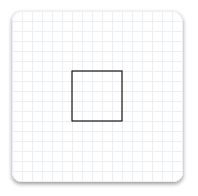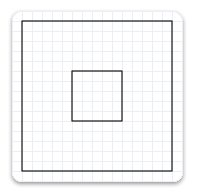# ID2D1TransformedGeometry interface (d2d1.h)

Represents a geometry that has been transformed.

## Inheritance

The ID2D1TransformedGeometry interface inherits from ID2D1Geometry. ID2D1TransformedGeometry also has these types of members:

## Methods

The ID2D1TransformedGeometry interface has these methods.

ID2D1TransformedGeometry::GetSourceGeometry

Retrieves the source geometry of this transformed geometry object.
ID2D1TransformedGeometry::GetTransform

Retrieves the matrix used to transform the ID2D1TransformedGeometry object's source geometry.

## Remarks

Using an ID2D1TransformedGeometry rather than transforming a geometry by using a render target's transform enables you to transform a geometry without transforming its stroke.

### Creating ID2D1TransformedGeometry Objects

To create an ID2D1TransformedGeometry, call the ID2D1Factory::CreateTransformedGeometry method.

Direct2D geometries are immutable and device-independent resources created by ID2D1Factory. In general, you should create geometries once and retain them for the life of the application, or until they need to be modified. For more information about device-independent and device-dependent resources, see the Resources Overview.

## Examples

The following example creates an ID2D1RectangleGeometry, then draws it without transforming it. It produces the output shown in the following illustration.``````hr = m_pD2DFactory->CreateRectangleGeometry(
D2D1::RectF(150.f, 150.f, 200.f, 200.f),
&m_pRectangleGeometry
);

``````

The next example uses the render target to scale the geometry by a factor of 3, then draws it. The following illustration shows the result of drawing the rectangle without the transform and with the transform; notices that the stroke is thicker after the transform, even though the stroke thickness is 1.``````// Transform the render target, then draw the rectangle geometry again.
m_pRenderTarget->SetTransform(
D2D1::Matrix3x2F::Scale(
D2D1::SizeF(3.f, 3.f),
D2D1::Point2F(175.f, 175.f))
);

m_pRenderTarget->DrawGeometry(m_pRectangleGeometry, m_pBlackBrush, 1);

``````

The next example uses the CreateTransformedGeometry method to scale the geometry by a factor of 3, then draws it. It produces the output shown in the following illustration. Notice that, although the rectangle is larger, its stroke hasn't increased.`````` // Create a geometry that is a scaled version
// of m_pRectangleGeometry.
// The new geometry is scaled by a factory of 3
// from the center of the geometry, (35, 35).

hr = m_pD2DFactory->CreateTransformedGeometry(
m_pRectangleGeometry,
D2D1::Matrix3x2F::Scale(
D2D1::SizeF(3.f, 3.f),
D2D1::Point2F(175.f, 175.f)),
&m_pTransformedGeometry
);

``````
``````// Replace the previous render target transform.
m_pRenderTarget->SetTransform(D2D1::Matrix3x2F::Identity());

// Draw the transformed geometry.
m_pRenderTarget->DrawGeometry(m_pTransformedGeometry, m_pBlackBrush, 1);

``````

## Requirements

Minimum supported client Windows 7, Windows Vista with SP2 and Platform Update for Windows Vista [desktop apps | UWP apps]
Minimum supported server Windows Server 2008 R2, Windows Server 2008 with SP2 and Platform Update for Windows Server 2008 [desktop apps | UWP apps]
Target Platform Windows